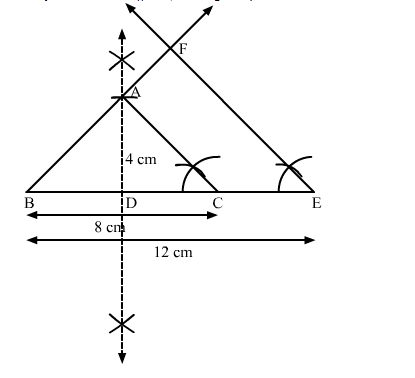# Construct an isosceles triangle whose base is 8 cm and altitude 4 cm`
Question:

Construct an isosceles triangle whose base is $8 \mathrm{~cm}$ and altitude $4 \mathrm{~cm}$ and then another triangle whose sides are $1 \frac{1}{2}$ times the corresponding sides of the isosceles triangle.

Solution:

Steps of Construction

Step 1. Draw a line segment BC = 8 cm.

Step 2. Draw the perpendicular bisector XY of BC, cutting BC at D.

Step 3. With D as centre and radius 4 cm, draw an arc cutting XY at A.

Step 4. Join AB and AC. Thus, an isosceles ∆ABC whose base is 8 cm and altitude 4 cm is obtained.

Step 5 . Extend $B C$ to $E$ such that $B E=\frac{3}{2} B C=\frac{3}{2} \times 8 \mathrm{~cm}=12 \mathrm{~cm}$.

Step 6. Draw EF || CA, cutting BA produced in F.Here, $\Delta \mathrm{BEF}$ is the required triangle similar to $\triangle \mathrm{ABC}$ such that each side of $\triangle \mathrm{BEF}$ is $1 \frac{1}{2}$ (or $\frac{3}{2}$ ) times the corresponding side of $\triangle \mathrm{ABC}$.